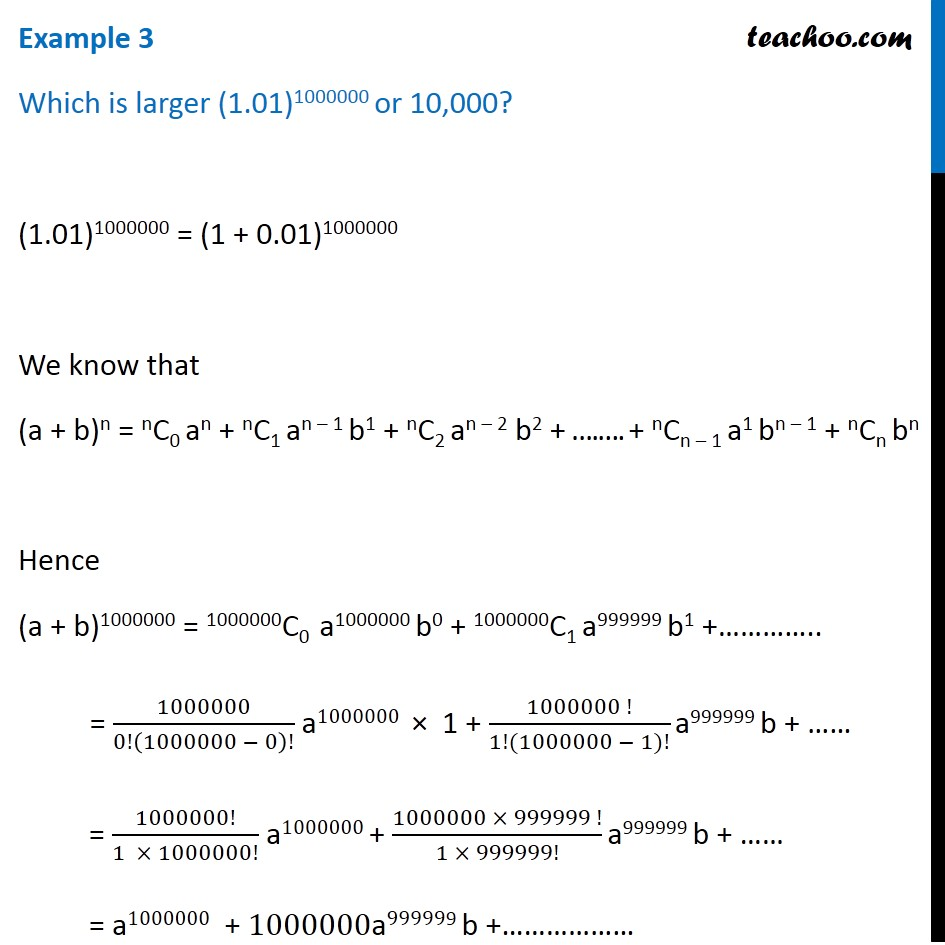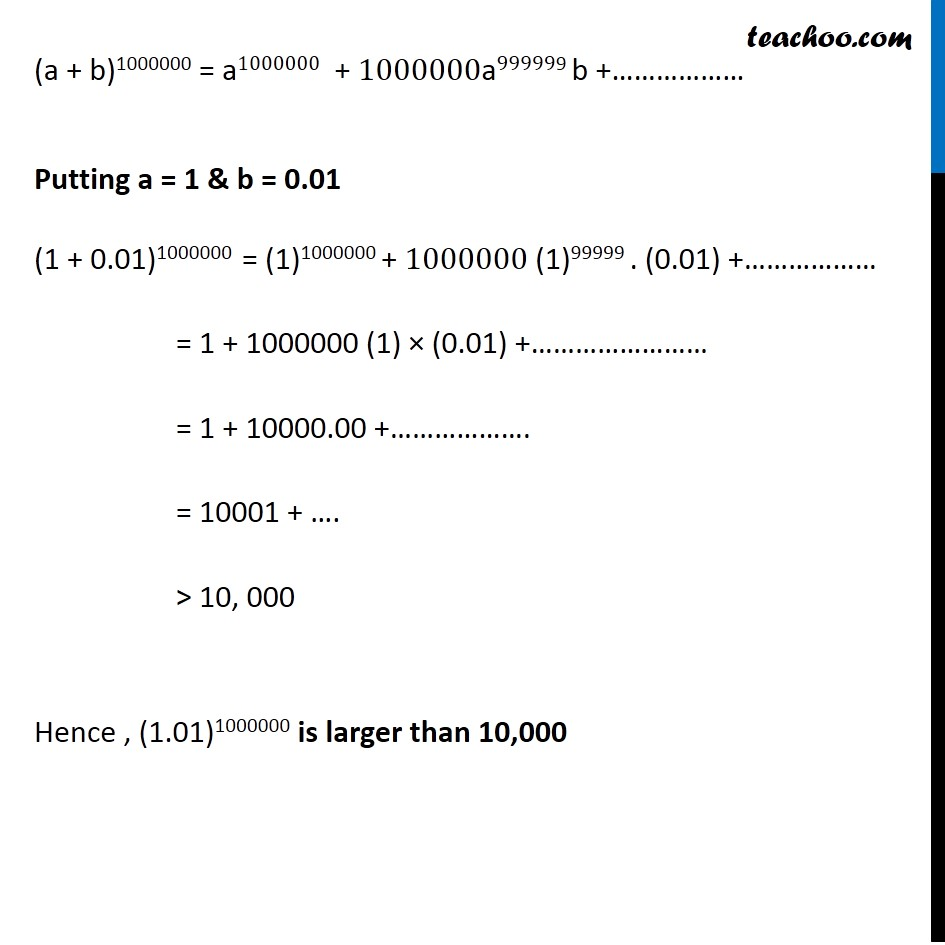Which is greater

Chapter 8 Class 11 Binomial Theorem
Concept wiseIntroducing your new favourite teacher - Teachoo Black, at only ₹83 per month

### Transcript

Example 3 Which is larger (1.01)1000000 or 10,000? (1.01)1000000 = (1 + 0.01)1000000 We know that (a + b)n = nC0 an + nC1 an – 1 b1 + nC2 an – 2 b2 + ….…. + nCn – 1 a1 bn – 1 + nCn bn Hence (a + b)1000000 = 1000000C0 a1000000 b0 + 1000000C1 a999999 b1 +………….. = 1000000/0!(1000000 − 0)! a1000000 × 1 + (1000000 !)/(1!(1000000 − 1)!) a999999 b + …… = 1000000!/(1 × 1000000!) a1000000 + (1000000 × 999999 !)/(1 × 999999!) a999999 b + …… = a1000000 + 1000000a999999 b +……………… (a + b)1000000 = a1000000 + 1000000a999999 b +……………… Putting a = 1 & b = 0.01 (1 + 0.01)1000000 = (1)1000000 + 1000000 (1)99999 . (0.01) +……………… = 1 + 1000000 (1) × (0.01) +…………………… = 1 + 10000.00 +………………. = 10001 + …. > 10, 000 Hence , (1.01)1000000 is larger than 10,000